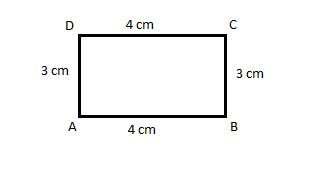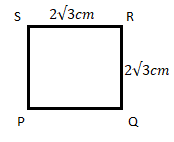Courses
Courses for Kids
Free study material
Offline Centres
MoreLast updated date: 05th Dec 2023
Total views: 382.5k
Views today: 4.82k

# Draw a rectangle of length 4 cm, breadth 3 cm and draw a square of the same area.Verified
382.5k+ views
Find the area of the rectangle using the length and breadth. Area of the square is equal to the area of the rectangle. Now using this area find the side of the square and draw the square.

Given the length and breadth of a rectangle, let us name the rectangle as ABCD.
Given length = 4 cm and breadth = 3 cm.

In a rectangle, opposite sides are parallel and opposite sides are equal in length.

Here, AB = CD = 4 cm length.

BC = DA = 3 cm breadth.

So let us draw the rectangle ABCD.We know the area of a rectangle is given by length multiplied by breadth.
Area of rectangle ABCD = length $\times$ breadth.
= 4 cm $\times$ 3 cm = 12$c{{m}^{2}}$
Now we have to draw a square with the same area as a rectangle.
Area of square = 12$c{{m}^{2}}$.
We know in a square all the sides are equal. Let the square be named as PQRS. Let us consider ‘a’ as the side of the square PQRS.
As all the sides are all, we can say that:
PQ = QR = RS = PS.
The area of the square is given by the formula of the square of its sides.
Area of square =${{(side)}^{2}}$.
Area of square =${{a}^{2}}$ .
We got the area of the square as 12$c{{m}^{2}}$. We need to find the side of a square PQRS.
${{a}^{2}}$ = 12.
a = $\sqrt{12}$ =$\sqrt{3\times 4}$.
a =$2\sqrt{3}cm$.
Therefore the side of the square =$2\sqrt{3}cm$.
I.e. PQ = QR = RS = PS =$2\sqrt{3}cm$.
Now let us draw the square PQRS with side$2\sqrt{3}cm$.Note: The side of the square can be calculated directly by equating the area of the rectangle to the area of the square. As it is mentioned in the question that both the areas are the same:
Area of rectangle = Area of square.
Length $\times$ breadth =${{(side)}^{2}}$.
4 $\times$ 3 =${{a}^{2}}$.
${{a}^{2}}$ = 12 cm.
a =$2\sqrt{3}cm$.